## conference papersJOURNAL OFAPPLIEDCRYSTALLOGRAPHY
ISSN: 1600-5767
Volume 40| Part s1| April 2007| Pages s235-s239

## Analysis of small-angle X-ray scattering data of protein–detergent complexes by singular value decomposition

aDepartment of Physics, Stanford University, Stanford, CA 94305, USA, bJoint Center for Structural Genomics and The Scripps Research Institute, Department of Molecular Biology, La Jolla, CA 92037,USA, cDepartment of Applied Physics, Stanford University, Stanford, CA 94305, USA, and dStanford Synchrotron Radiation Laboratory, Stanford University, Stanford, CA 94305, USA
*Correspondence e-mail: doniach@drizzle.stanford.edu

(Received 16 August 2006; accepted 2 February 2007; online 3 March 2007)

Small-angle X-ray scattering can be a valuable tool in the structural characterization of membrane protein–detergent complexes (PDCs). However, a major challenge is to separate the PDC scattering signal from that of the empty' detergent micelle in a protein–detergent mixture. We briefly review an approach that allows approximate determination of the PDC scattering signal at low momentum transfer and present a novel approach that employs a singular value decomposition (SVD) and fitting of scattering data collected at different protein–detergent stoichiometries. The SVD approach allows the scattering profile for the PDC over the entire measured momentum transfer range to be obtained, it is applicable to strongly scattering detergents and can take into account interparticle interference. The two approaches are contrasted and an application to the membrane protein TM0026 from Thermotoga maritima is presented.

### 1. Introduction

Membrane proteins are located in the cell membrane, where they act as transporters, channels, receptors or enzymes in a range of essential cellular functions. An estimated 30–40% of all genes code for mebrane proteins (Wallin & von Heijne, 1998) and they constitute ca 50% of all drug targets (Korepanova et al., 2005). In contrast, less than 1% of the structures currently deposited in the Protein Data Bank (Berman et al., 2000) are of membrane proteins. A major hurdle to structural studies is the necessity to solubilize membrane proteins (Sanders & Sonnichsen, 2006). Micelle forming detergents are routinely used as a mimetic of the cell membrane. The hydrophobic regions of the protein are encapsulated on the inside of the detergent micelle in the resulting protein–detergent complex (PDC).

Insights into the structure of the PDC provide information about the structure of the membrane protein itself, and, furthermore, about protein–detergent interactions. An improved understanding of protein–detergent interactions can be used to choose an optimal detergent for a given protein and application. Finally, knowledge about the interactions of PDCs in solution could help to design better crystallization conditions.

Small-angle X-ray scattering (SAXS) can serve as a powerful probe of the PDC, as it can probe the size, shape and interactions of macromolecular complexes under a variety of solution conditions without the need to crystallize the sample (Doniach, 2001; Svergun & Koch, 2003; Koch et al., 2003). The scattering signal from a mixture of membrane proteins and detergent, however, generally contains contributions both from the PDC and from empty' detergent micelles. A major challenge is to separate the contributions and to isolate the scattering profile of the PDC.

One apparent possibility is to record a scattering profile of the detergent only, i.e. in the absence of protein, for subtraction of the micelle signal. However, as an (a priori unknown) fraction of the detergent molecules form PDCs in the presence of protein, the empty' micelle concentration is different in the absence and presence of the protein and the subtraction will be mismatched (see §3.1). Loll et al. (2001) performed light scattering studies after extensive dialysis against a buffer of known detergent concentration to ensure a fixed concentration of detergent micelles. This approach is problematic, however, as for each PDC dialysis the conditions need to be optimized and several days of dialysis are required. An alternative strategy is to match the scattering density of the buffer to that of the detergent, such that the detergent molecules become invisible' to the scattering experiment. Bu & Engelman (1999) followed this approach and used sucrose solutions of different concentrations for density matching to determine the radius of gyration and molecular weight of a model protein system. However, matching the electron density in this way is only possible for a select few detergents that have a scattering density close to that of water, as the scattering contrast for X-ray scattering (i.e. the electron density) is difficult to adjust. This is unlike the case of neutron scattering experiments, where the buffer scattering contrast can be changed over a wide range by adjusting the ratio of D2O to H2O (Knoll et al., 1981).

Here, we present two approaches to deconvolving micelle and PDC scattering. First we briefly review a recently developed approximative expansion' treatment (Columbus et al., 2006) that can be used to obtain an upper and lower limit of the PDC scattering in the low-angle Guinier region from a single measurement of the protein sample. This approximative treatment is well suited to obtaining the radius of gyration and protein oligomerization state of the PDCs for a large number of protein–detergent combinations in a high-throughput fashion, however, it suffers several important shortcomings.

We then present a novel method to deconvolve the PDC and micelle scattering based on a singular value decomposition (SVD) of scattering data collected at different protein–detergent stoichiometries. Finally, we demonstrate the feasibility of the SVD approach by applying it to scattering data for the integral membrane protein TM0026 from Thermotoga maritima. The results are compared with the approximative approach and the relative advantages of the methods are discussed.

### 2. SAXS measurements

Membrane proteins were expressed and purified as described in Columbus et al. (2006). All data were obtained at beamline 12-ID of the Advanced Photon Source at Argonne National Laboratory, USA, using a set-up as described in Lipfert, Millet et al. (2006), Beno et al. (2001) and Seifert et al. (2000). For each data point, a total of ten measurements of 0.1 s integration time each were taken. Data were image corrected and circularly averaged; the ten profiles for each condition were averaged to improve signal quality.

### 3. Theory

#### 3.1. Expansion' treatment of the PDC scattering signal

Recently, we have developed a method to obtain the PDC forward scattering intensity I(0) and radius of gyration Rg approximatively (Columbus et al., 2006) from Guinier analysis (Guinier, 1939). The basic idea is to consider two different estimates of the PDC scattering profile: In one limit, referred to as I(complex − buffer), we consider the scattering from the protein–detergent mixture and subtract a suitable buffer (no detergent) profile. This provides an overestimate of the PDC scattering signal, as the contribution of the empty' detergent micelles is not subtracted. The I(complex − buffer) limit yields an upper bound of the PDC forward scattering intensity and a lower bound of the PDC Rg (Columbus et al., 2006). Denoting the empty' micelle scattering intensity with Imic, that of the PDC with IPDC, the protein concentration with cprot and that of the empty' detergent micelles in the presence of the protein with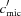, we introduce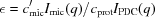. The I(complex − buffer) limit provides a good approximation for small, i.e. whenever the scattering signal of the micelles is weak compared to the PDC scattering and for small concentrations of empty' detergent micelles. In the other limit, denoted as I(complex − micelle), we subtract the micelle scattering profile recorded at the same detergent concentration from the scattering of the protein–detergent mixture. As the concentration of micelles in the absence of protein cmic is larger than the the micelle concentration in the presence of protein, i.e.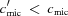, this provides a lower bound for the PDC forward scattering and an upper bound of the PDC Rg. The I(complex − micelle) limit provides a good approximation for small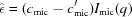/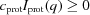, i.e. for weakly scattering micelles and in the limit that the detergent is in large excess over the protein, such that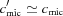.

We have applied this approximative treatment in a screen of eight integral membrane proteins from Thermotoga maritima and 11 different detergents (Columbus et al., 2006). For many of the studied protein–detergent pairs the upper and lower bounds for Rg and I(0) from the two different expansions were close and we were able to determine the Rg and oligomerization state of the protein with reasonable accuracy. An advantage of this approximative treatment is that it only requires a single measurement of the protein sample (and two measurements of the buffer' and detergent only' solutions).

However, this approximative approach suffers several important shortcomings. It is inapplicable or provides poor estimates for strongly scattering detergent micelles. Out of the 11 detergents employed in our recent study (Columbus et al., 2006), we found in particular n-decyl-β-D-maltoside (DM) and n-dodecyl-β-D-maltoside (DoDM) and to a lesser extent 3-[(3-cholamidopropyl)dimethyl­ammonio]-1-propane sulfonate (CHAPS) to be strong scatterers. Even for weakly scattering detergents, a good estimate is only obtained for the PDC scattering at very low momentum transfer q [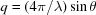, where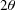is the total scattering angle and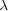is the X-ray wavelength]. As the PDC is typically much larger and more electron dense than the empty' micelles, it scatters more strongly at low q. Micelle scattering, however, typically exhibits a strong second peak at intermediate to high q, which results from the interference between the electron dense detergent head groups and the low density aliphatic tail groups in the middle of the micelle. The high-q micelle scattering, therefore, typically exceeds that of the PDC even for weakly scattering detergents. Finally, the approximative treatment neglects interparticle interference effects, and is therefore strictly speaking only applicable to very dilute solutions.

#### 3.2. Analysis by singular value decomposition

We will now present a method that can overcome some of the shortcomings of the approximative expansion' approach. It requires that scattering data are collected at several different protein–detergent stoichiometries. In return, it allows the scattering profile for the PDC over the entire measured angle range to be obtained, it is applicable to strongly scattering detergents and can take into account interparticle interference.

Consider K scattering profiles collected at different protein and detergent concentrations (cprot,k, cdet,k). The data can be arranged in a matrix A, where the rows correspond to different momentum transfer values qj and the columns are the intensity profile for the kth condition. Applying a singular value decomposition to the data matrix deconvolves the signal into a set of orthogonal basis functions as follows (Henry & Hofrichter, 1992; Segel et al., 1998; Doniach, 2001)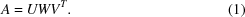For the case of N discrete momentum transfer values, A is an N × K matrix. The matrix U is also N × K and has as its columns orthogonal basis functions Ui(qj) ( = Uj,i). W is a K × K diagonal matrix containing the singular values wi on the main diagonal. The singular values are ordered, i.e. they have the property that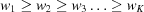. Following Henry & Hofrichter (1992), the number of true, independent basis functions Ui(q) corresponds to the number of distinctly scattering species L. For homogeneous populations of micelles and PDCs, we would expect L = 2 independently scattering components. However, in practice it is necessary to include more components into the subsequent fitting to account for the effects of interparticle interference.

#### 3.3. Interparticle interference

For approximately spherical particles in solution, the total scattering intensity () is a product of the concentration-independent form factor I(q) [often also denoted P(q)], which represents the scattering signal from a single particle at infinite dilution and the structure factor S(q,c), which depends in the concentration c and takes into account interparticle effects.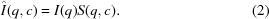In the limit of infinite dilution S(q,c) = c, independent of q, and the scattering intensity is linear in the concentration. We account for interference by expanding S(q,c) in powers of c. Keeping the linear and quadratic term in concentration, the scattering for two molecular species, the PDC and detergent micelle, reads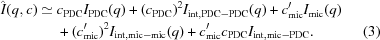The interference is taken into account by introducing interference components' Iint, PDC-PDC, Iint, mic-mic and Iint, mic-PDC in addition to the particle form factor scattering components' IPDC and Imic. In principle, it is possible to take into account interference effects to higher order by introducing additional interference components.

#### 3.4. Number of independent components

The number of signal-containing basis functions Ui(q) determines how many scattering' and interference' components should be used in the fit. Henry & Hofrichter (1992) suggest the following three criteria to determine the number L of signal-containing components: (1) Inspection of the basis functions: by plotting the basis functions Ui(q) as a function of q, one can can estimate which of the Ui(q) contain appreciable levels of signal and which components correspond to noise. (2) Singular values: the size of the singular values gives an estimate of the relative importance of the corresponding basis components. (3) Autocorrelations of the basis functions: by computing the autocorrelation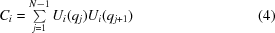of each of the basis functions, an estimate of the noisiness' is obtained. Components which contain appreciable signal typically have autocorrelations close to 1.0 (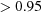), whereas components that correspond to noise tend to have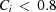.

The assignment of the independent components to molecular species or interference components' requires modeling assumptions and must be guided by prior knowledge.

In the following, we treat the case of four independent components: IPDC, Imic, as well as two interference components' Iint, mic-mic(q) and Iint, mic-PDC(q), i.e. we neglect IPDC-PDC in equation (3)(see below). A generalization of the method to more (or fewer) scattering components is straightforward. Fitting of L independent components requires measurements of at least K = L different protein–detergent mixtures. In practice, it is desirable to have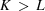experimental profiles.

#### 3.5. Thermodynamic model

From the known concentrations of protein and detergent for the K stoichiometries (cprot,k, cdet,k), we estimate the concentration of micelles in the presence of protein as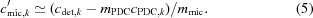Out of the total number of detergent molecules cdet, mPDC cPDC participate in PDCs, i.e. each PDC contains one protein and mPDC detergent monomers. The remaining detergent molecules form empty' micelles of aggregation number mmic. This assumes that the protein is monomeric inside the PDC, i.e. that cPDC = cprot. The oligomerization state of the protein inside the PDC can be obtained from the approximative expansion' treatment or from e.g. chemical cross-linking experiments (Columbus et al., 2006). For higher protein oligomers cprot is to be divided by the appropriate factor.

We neglect free detergent monomers (i.e. monomers that neither participate in micelles nor in PDCs). The concentration of free detergent is of the order of the critical micelle concentration, which is typically much lower than the detergent concentrations used in our experiments. Furthermore, we neglect the weak dependence of micelle size and aggregation number on detergent concentration (empirically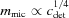; Quina et al., 1995). We also found in a recent study that the dominant effect on the scattering profile for DM with increasing detergent concentration is interparticle interference, and not micelle growth (Lipfert, Columbus et al., 2006). Values for mmic are available for many detergents from the literature or can be determined from Guinier analysis of the detergent forward scattering intensity (Lipfert, Columbus et al., 2006). mmic needs to be only approximately known, see below. The parameter mPDC is determined from the fit.

#### 3.6. Fitting to the SVD data

The data matrix Aj,k with columns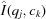can be approximated by the first L components Ui(q), with the weights given by the SVD as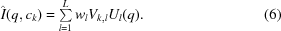It is a general property of the SVD that equation (6)is the best approximation of the data in the least-squares sense for any set of L vectors (Golub & Van Loan, 1996). As the Ui(q) form a linear independent basis set, the (yet to be determined) scattering profiles Imic(q) and IPDC(q) as well as Iint,mic-mic(q) and Iint,mic-PDC(q) can be written as linear combinations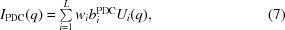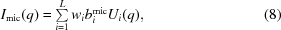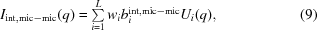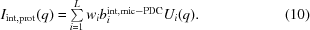The coefficients biPDC, bimic, biint,mic-mic and biint,mic-PDC are to be determined by the fitting procedure.

Combining equations (7–10)with (3)and comparing coefficients component by component (as the Ui are linearly independent) with equation (6)we find that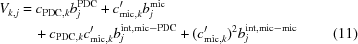for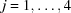. We can employ a nonlinear fitting routine in order to determine the coefficients bi as well as the aggregation number mPDC to obtain an optimal fit to the data by minimizing the function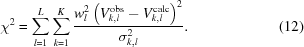Here the Vk,lobs are the coefficients obtained from the SVD of the data matrix [equation (6)] and the Vk,lcalc are the modeled coefficients from equation (11). The errors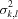are the variances of the coefficients from the SVD and are simple linear combinations of the experimental errors (Henry & Hofrichter, 1992). We used a nonlinear fitting routine implemented in Matlab (Mathworks) to obtain fits to the SVD data from our model. The aggregation number of the free micelle mmic in equation (5)is not a free parameter, as it simply sets the scale of the bimic and biint,mic-mic. However, we need to ensure that the numerical value of mmic is such that the concentrationsare smaller than unity.

### 4. Results

Scattering data for the membrane protein TM0026 in n-decyl-β-D-maltoside (DM) were obtained as described in §2. TM0026 is an-helical protein with two predicted transmembrane helices and a molecular weight of 9.6 kDa. Judging from one- and two-dimensional nuclear magnetic resonance and circular dichroism spectroscopy, TM0026 is well folded and does not aggregate when solubilized in DM micelles (Columbus et al., 2006). Using the expansion' approach, it was determined to be monomeric in PDCs formed by five different detergents, including DM (Columbus et al., 2006).

For this work, a total of six scattering profiles were collected, three at a protein concentration of 0.18 mM and detergent concentrations of 88, 150 and 300 mM, another three at identical detergent concentrations and a protein concentration of 0.36 mM. All measurements were performed in 20 mM phosphate buffer, pH 7.0, with 150 mM NaCl added. Scattering profiles of this buffer were subtracted for background correction.

We determine the number of signal-containing components by applying the criteria of Henry & Hofrichter (1992). Fig. 1shows the first five basis components Ui(q) obtained from an SVD of the scattering data matrix. The plots of the basis functions suggest that the first four components contain significant signal, whereas the fifth (and sixth, not shown) are representative of noise. This finding is corroborated by the autocorrelations computed from equation (4), which are found to be 0.99, 0.99, 0.97, 0.94, 0.63 and 0.49 for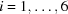. The first four components have autocorrelations of 94% and higher, while the last two components exhibit much lower values.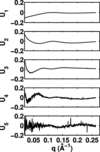Figure 1 The first five basis functions Ui(q) from an SVD of SAXS data of the membrane protein TM0026 in DM at six different stoichiometries (see text). The momentum transfer q is equal to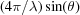, whereis the total scattering angle andis the X-ray wavelength.

With the number of signal-containing components determined to be L = 4, we fit IPDC(q), Imic(q) and two interference components' to the data. As the aggregation number for DM is ~70 (Sigma Aldrich, 2004), the micelle concentration is higher than the PDC concentration for all experimental stoichiometries. Therefore, we neglect the (cPDC)2 term in equation (3)and fit Iint, mic-mic and Iint, mic-PDC as interference components. This approach is further corroborated by the fact that scattering data collected on DM detergent micelles for DM concentrations ranging from 5 to 200 mM yield two signal-containing components (data not shown).

The IPDC(q), Imic(q) and two interference profiles' obtained from the best fit to the data are shown in Fig. 2. The number of detergent monomers in the PDC was fitted to be ~100–120. Interestingly, this value is larger than the aggregation number of the empty micelle, which suggests that the detergent packing is significantly perturbed in the PDC as compared to the micelle. By Guinier analysis of the fitted IPDC(q) and Imic(q), the radius of gyration of the PDC was determined to be 40 Å, that of the micelle to be 27 Å. Using the expansion' approach, we had previously only been able to bracket the Rg of the TM0026–DM PDC coarsely as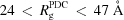, as DM is a strongly scattering detergent (Columbus et al., 2006). The value of 27 Å for the micelle Rg is in excellent agreement with the value of 27 ± 0.5 Å determined from direct measurements of detergent scattering (Lipfert, Columbus et al., 2006). The fitted scattering profile Imic(q) agrees well with the measured scattering profiles for empty' DM micelles (not shown). Overall, the fit to the data is excellent, as shown in Fig. 3.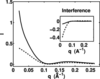Figure 2 Scattering profiles and interference profiles for TM0026 in DM obtained from fitting to the SVD data. Main graph: IPDC(q) (solid line) and Imic(q) (dashed line). Inset: Interference components Iint, mic-PDC(q) (solid line) and Iint, mic-mic(q) (dashed line).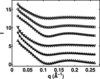Figure 3 Experimental SAXS profiles for TM0026 in DM (symbols) and fitted profiles from the SVD (solid lines). The protein detergent and concentrations are, from top to bottom, (cprot =) 0.18 mM, (cdet =) 88 mM (diamonds), 0.18, 150 mM (circles), 0.18, 300 mM (squares), 0.36, 88 mM (downwards pointing triangles), 0.36, 150 mM (stars), 0.36, 300 mM (upwards pointing triangles). For clarity, the number of experimental momentum transfer values was reduced and the profiles were vertically offset.

The fitted interference components (inset of Fig. 2) quickly go to zero for high q, as is to be expected as generally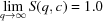. For low q values they are negative, characteristic of interparticle repulsion. As DM is a non-ionic detergent, this repulsion is likely to be due to excluded volume effects.

### 5. Conclusion

We have shown that the scattering profile of the PDC can be separated from the micelle scattering by using SVD analysis and fitting to data of protein–detergent mixtures at different stoichiometries. This approach, in contrast to the approximative `expansion' treatment, requires measurements of several protein samples, which makes it less well suited to high-throughput data collection. In return, it allows the reconstruction of the scattering profile for the PDC over the entire measured q range, which is advantageous for subsequent modeling of the PDC. The SVD approach is applicable even to strongly scattering detergents; furthermore, interparticle interference can be taken into account.

### Acknowledgements

We thank Sönke Seifert for help with data collection at the APS. This research was supported by the National Science Foundation Grant PHY-0140140, and the National Institutes of Health Grant PO1 GM0066275. Use of the Advanced Photon Source was supported by the US Department of Energy, Office of Science, Office of Basic Energy Sciences, under Contract No. W-31-109-Eng-38.

### ReferencesJOURNAL OFAPPLIEDCRYSTALLOGRAPHY